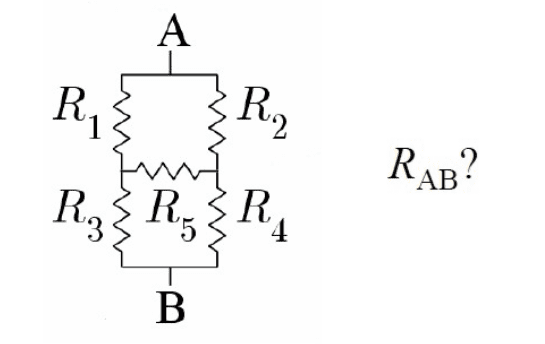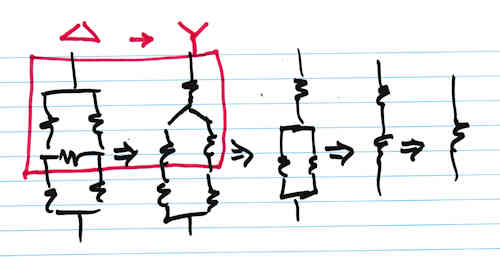# Find the equivalent resistance of this 5-resistor circuit

• aaronll
Superposition on nodes" , because with that is totally clear.In summary, the conversation is about a student asking for help with a circuit problem and the expert providing guidance on how to use Kirchhoff's laws and delta-Y transforms to solve it. The expert also suggests using the superposition method to simplify the equations. f

#### aaronll

I have a question about an exercise I don't understand, I don't want the solution, only the first element to begin.I have tried with kirchhoff law, but I failed.
thanks

Remember that you need to show some effort on schoolwork-type problems. If there are certain symmetries in this bridge circuit, you can sometimes simplify it. But in general you would use KCL or similar to solve it. Can you show us how to use KCL to solve it?

•ammarb32
Remember that you need to show some effort on schoolwork-type problems. If there are certain symmetries in this bridge circuit, you can sometimes simplify it. But in general you would use KCL or similar to solve it. Can you show us how to use KCL to solve it?
If I start with these equation, assuming current I_i for i = 1,2,3,4,5, and using KCL and what I know about circuit and correlated laws:
I have:

I_1 + I_2 = I_{tot}
I_1 = I_5 + I_3
I_2 + I_5 = I_4
I_3 + I_4 = I _{tot}

and for general I have:
## R_{tot} = \frac{V_{AB}}{I_{tot}}##

with the equation:

R_1 * I_1 + R_3 * I_3 = V_{AB}
and so on, for total of 4 equation, each equal to V_{AB}

By now, is correct my resolution?
to me seem very strange

P.S. excuse for some LaTex code but seem not work, If you help me also with this :)

Last edited:
What you have written is correct (although I would give points off for your failure to SHOW the currents even though it's pretty clear that you are using a reasonable convention). BUT ... what does that have to do with the equivalent resistance? You are not asked to give a bunch of equations, you are asked to give ONE equation of the form

$R_{eq}$ = <whatever>

What you have written is correct (although I would give points off for your failure to SHOW the currents even though it's pretty clear that you are using a reasonable convention). BUT ... what does that have to do with the equivalent resistance? You are not asked to give a bunch of equations, you are asked to give ONE equation of the form

$R_{eq}$ = <whatever>
Yes, ##R_{tot}## that I have written is the ##R_{eq}##, but to find that resistance... I tried with KCL with that bunch of equation, but I don't arrive at the end because there always too much variable in the equation I found ( e.g. for replacement)

Do you know about delta-Y transforms?

•FactChecker
Do you know about delta-Y transforms?
According to my professor they come later.. so first I should able to resolve this exercise, but I don't understand how

According to my professor they come later.. so first I should able to resolve this exercise, but I don't understand how
Well, since you can't just USE the transform equations, it is now up to you to derive them. This will allow you to break your problem into two parts --- one fairly messy, the transform equations, and the other very simple conceptually but still involving messy equations in this case because you have so many unknowns.

Take the upper 3 resistors as a delta and convert that to a Y. That's the hard part. Then you have a very simple series/parallel circuit that is algebraically trivial but which will look messy as all get-out in this case because of the 5 unknowns you have to carry around.

The progression will look like this:Literally speaking, the delta and Y are upside down in this case, but they are still delta and Y in electronics.

•FactChecker and aaronll
I should add, by the way, that there is usually more than one way to solve simple circuits like this and I've just given you my way because I think it's the easiest but that's 'cause I'm used to delta-Y transforms.

•aaronll
Finally I understand how to find the equivalent resistance with the delta - star transformation... but it was sufficent to say "Superposition on nodes" , because with that is totally clear.
Anyway thanks for the help.

Yes, ##R_{tot}## that I have written is the ##R_{eq}##, but to find that resistance... I tried with KCL with that bunch of equation, but I don't arrive at the end because there always too much variable in the equation I found ( e.g. for replacement)
I think the question was asking you, prompting you, to define, that is to write out the equation for equivalent resistance. Just for the sake of a logical and suggestive order of procedure.

Then this definition contained so it's related to things you have already written and you can start to fill in, substitute et cetera. As I said, just one way of proceeding there are more

Last edited:
Finally I understand how to find the equivalent resistance with the delta - star transformation... but it was sufficent to say "Superposition on nodes" , because with that is totally clear.
Anyway thanks for the help.
Can you show us how you did it with "Superposition on nodes" so that when other people search for a solution to this problem in the future they will find your work?

•SammyS